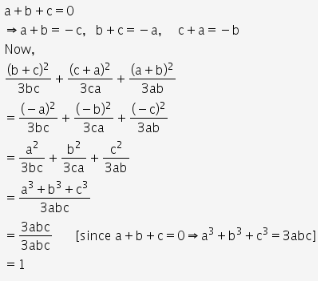# If a+b+c = 0, then prove that (b+c)^2 / 3bc + (c+a)^2 / 3ca + (a+b)^2 / 3ab = 1

If a+b+c = 0, then prove that (b+c)2 / 3bc + (c+a)2 / 3ca + (a+b)2 / 3ab = 1